PHY294H - Lecture 13

Recommended problems: FGT 26.62, 22.65, 22.69
Recommended reading for Lecture 14: Chap. 27 FGT

Dielectric materials (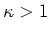)

In order to treat the effect of materials' structure on the electrostatic response of materials, we need to know two properties of the materials. The first and most important is the dielectric constant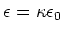and the second is the threshold field at which the material fails'' and begins to conduct, Ec. For insulators Ec>0while for conductors Ec=0. Insulators which are used in capacitors and other applications requiring electrical insulation are often called dielectrics. In practice, there is a simple way to include the effect of dielectric properties of materials on electrostatic response we simply make the replacement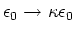in all of our electrostatic formula. For example Coulomb's law is now,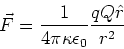(1)

Notice that dielectric materials have the property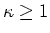which reduces the force between two point charges. This is due to screening'' by the charge structure of the material and this screening is more effective the stronger the dielectric response of the materials. As another example, consider the magnitude of the electric field between two plates with equal and opposite charge densities,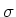,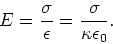(2)

The electric field between the parallel plates is reduced. The capacitance is defined by Q=CV. Since the electric field is reduced when, so is the potential. Therefore we expect the capacitance to increase. This is evident in the formula,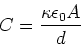(3)

We thus see that dielectric materials (which have) reduce the Coulomb force, reduce the electric field, reduce the electric potential and the electric potential energy and increase the capacitance.

Example: 26-7 of FGT

A parallel plate capacitor has area A=20cm2 and plate separation d=4mm. Find its capacitance if the gap is filled with: a) Air (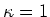) b) Teflon (). Now consider the following experiment. Use a battery to apply a voltage of 24V to the capacitor with an air gap. Now disconnect the battery. Then insert the Teflon dielectric into the gap. Find the stored potential energy before and after insertion of the Teflon. Where has the energy gone?

a)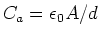b)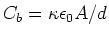c) The amount of charge on the plates of the capacitor is Q = Ca Va. This capacitor with the air gap thus stores energy Q2/2Ca. When the battery is disconnected and the Teflon inserted, the charge remains the same, but the voltage is now given by, Q=Cb Vb. This capacitor stores energy Q2/2Cb. Since Cb>Ca the stored potential energy decreases when the teflon replaces the air gap. In practice, this means that the dielectric material is sucked into'' the gap between the plates. Negative external work is required. This means that the capacitor itself does positive work.

Example: 26.8 of FGT

A parallel plate capacitor is disconnected and carries a charge of q=1nC. It has a gap which is d=4mm wide and contains a teflon sheet which is 2mm=d/2 thick. What is the electric field between the gap a) inside the teflon. b) inside the air within the gap. Now calculate the potential drop across the air gap and the teflon. Finally, find the capacitance of the system.

a) The charge on each plate is q. The electric field is stillinside the air.

b) Inside the teflon, the electric field has magnitude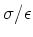.

c) The potential drop across the air is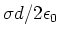. The potential drop across the teflon is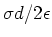.

d) The capacitance of the system is found from the definition q = CV. Where(4)

Dielectric materials - It's the dipoles stupid!

Dielectric materials can be considered to be composed of dipoles, either permanent dipoles or induced dipoles. We shall discuss the case of two plates which carry equal and opposite charges Q. These plates have a dielectric material between them and we want to understand the behavior of the dipoles in this material in the presence of the electric field produced by the plates of charge.

The dipoles in the dielectric material align with the electric field produced by the plates. This screens the charge on each of the plates of the capacitor. If Q is the true magnitude of charge on each plate, we introduce Qi to be the screening charge of the dipoles produced by the electric field. The charge that remains to generate the electric field between the plates is Q-Qi. The electric field between the plates is then,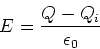(5)

The voltage between the plates is V = Ed where d is the plate separation. The capacitance is defined in terms of the true charge on the plates Q, so Q=CV. However the capacitance is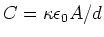. From this we want to find the induced charge Qi. From Eq. (5), we have,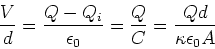(6)

From this we find,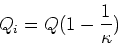(7)

We could also have found this directly from Eq. (5) by setting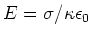. When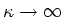, there is perfect screening of the electric field.

More on dielectrics

1. Polar (those with permanent dipole moments) dielectrics should line up more readily as the temperature is reduced. In many cases they obey K = 1 + c/T, where cis a constant. Polar dielectrics can sometimes show ferroelectricity. This is like magnetism but the spins are replaced by electric dipoles. In that case there is a critical temperature at which the dipoles spontaneously align.

2. The polarization of solids with permanent dipoles can sometimes be changed by applied stress. This can lead to a detectable electrical signal. Materials with this property are called piezoelectrics. Sometimes this property is used in reverse, ie. an electrical signal applied to piezo-electric can lead to a change in the shape of the material (e.g. quartz, BaTiO3...). This can be used to provide very sensitive control of movement and devices of this sort are called piezo-actuators. Piezo actuators are very important in nano-engineeing.